Updating search results...

# 27 Results

View
Selected filters:
• MI.Math.Content.3.NF.A.2Conditional Remix & Share Permitted
CC BY-NC-SA
Rating
0.0 stars

This task gives students the opportunity to analyze two number lines in order to identify the one that correctly shows an improper fraction. Students then communicate their understanding by describing the reasoning they used to determine their answer was correct. It is aligned to evidence statement 3.C.6-1

Subject:
Mathematics
Material Type:
Activity/Lab
Assessment
Homework/Assignment
Author:
Chelsea Davies
Linda Schoenbrodt
08/04/2020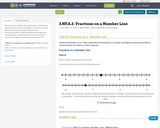Conditional Remix & Share Permitted
CC BY-NC-SA
Rating
0.0 stars

Fraction_Number_Lines.JPGTwo number lines are shown. The first number line shows the numbers 0 through 3 and is partitioned into 6 equal parts in each whole.

The second number line shows the numbers 0 through 3 and is partitioned into 8 equal parts in each whole.

Subject:
Mathematics
Material Type:
Activity/Lab
Homework/Assignment
Author:
Linda Schoenbrodt
Chelsea Davies
08/06/2020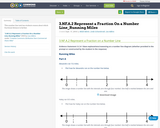Conditional Remix & Share Permitted
CC BY-NC-SA
Rating
0.0 stars

one_half.PNGThis image shows a number line with the intervals zero through four marked. One-half is marked between the zero and one.

Subject:
Mathematics
Material Type:
Activity/Lab
Homework/Assignment
Author:
08/06/2020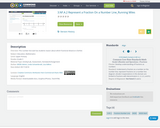Conditional Remix & Share Permitted
CC BY-NC-SA
Rating
0.0 stars

This number line task has students reason about which fractional distance is farther.

Subject:
Mathematics
Material Type:
Activity/Lab
Assessment
Homework/Assignment
Author:
Linda Schoenbrodt
08/04/2020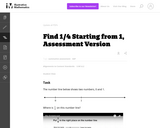Unrestricted Use
CC BY
Rating
0.0 stars

This is a task from the Illustrative Mathematics website that is one part of a complete illustration of the standard to which it is aligned. Each task has at least one solution and some commentary that addresses important asects of the task and its potential use. Here are the first few lines of the commentary for this task: The number line below shows two numbers, 0 and 1. Where is $\frac14$ on this number line? http://www.youtube.com/watch?v=HJ0LDgxVGfU...

Subject:
Mathematics
Material Type:
Activity/Lab
Provider:
Illustrative Mathematics
Provider Set:
Illustrative Mathematics
Author:
Illustrative Mathematics
03/18/2013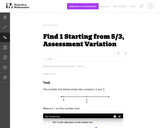Unrestricted Use
CC BY
Rating
0.0 stars

This is a task from the Illustrative Mathematics website that is one part of a complete illustration of the standard to which it is aligned. Each task has at least one solution and some commentary that addresses important asects of the task and its potential use. Here are the first few lines of the commentary for this task: The number line below shows two numbers, 0 and $\frac53$. Where is 1 on this number line? http://www.youtube.com/watch?v=wAXRjMUgiu4...

Subject:
Mathematics
Material Type:
Activity/Lab
Provider:
Illustrative Mathematics
Provider Set:
Illustrative Mathematics
Author:
Illustrative Mathematics
03/18/2013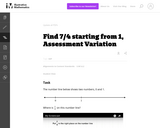Unrestricted Use
CC BY
Rating
0.0 stars

This is a task from the Illustrative Mathematics website that is one part of a complete illustration of the standard to which it is aligned. Each task has at least one solution and some commentary that addresses important asects of the task and its potential use. Here are the first few lines of the commentary for this task: The number line below shows two numbers, 0 and 1. Where is $\frac74$ on this number line? http://www.youtube.com/watch?v=TEzH_PbHZIw...

Subject:
Mathematics
Material Type:
Activity/Lab
Provider:
Illustrative Mathematics
Provider Set:
Illustrative Mathematics
Author:
Illustrative Mathematics
03/18/2013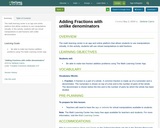Unrestricted Use
CC BY
Rating
0.0 stars

The math learning center is an app and online platform that allows students to use manipulatives virtually. In this activity, students will use virtual manipulatives to add fractions with unlike denominators

Subject:
Mathematics
Material Type:
Activity/Lab
08/28/2020Rating
0.0 stars

Students will use the Census Business Builder: Small Business Edition data access tool to gather and analyze information that entrepreneurs may consider when opening a business. This introductory activity assumes that students have limited experience using data access tools.

Subject:
Mathematics
Statistics and Probability
Material Type:
Activity/Lab
Provider:
U.S. Census Bureau
Provider Set:
Statistics in Schools
11/15/2019Conditional Remix & Share Permitted
CC BY-NC-SA
Rating
0.0 stars

In this video segment from Cyberchase, the CyberSquad must locate a crystal with the highest fraction of orange color.

Subject:
Mathematics
Material Type:
Lecture
Author:
WNET
U.S. Department of Education
08/05/2020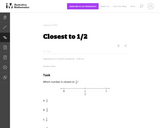Unrestricted Use
CC BY
Rating
0.0 stars

In this task using a number line, students must partition the interval between 0 and 1 into eighths.

Subject:
Mathematics
Numbers and Operations
Material Type:
Activity/Lab
Provider:
Illustrative Mathematics
Provider Set:
Illustrative Mathematics
Author:
Illustrative Mathematics
05/01/2012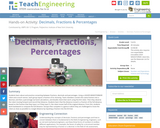Educational Use
Rating
0.0 stars

Students learn about and practice converting between fractions, decimals and percentages. Using a LEGO® MINDSTORMS® NXT robot and a touch sensor, each group inputs a fraction of its choosing. Team members convert this same fraction into a decimal, and then a percentage via hand calculations, and double check their work using the NXT robot. Then they observe the robot moving forward and record that distance. Students learn that the distance moved is a fraction of the full distance, based on the fraction that they input, so if they input ½, the robot moves half of the original distance. From this, students work backwards to compute the full distance. Groups then compete in a game in which they are challenged to move the robot as close as possible to a target distance by inputting a fraction into the NXT bot.

Subject:
Applied Science
Engineering
Mathematics
Material Type:
Activity/Lab
Provider:
TeachEngineering
Provider Set:
TeachEngineering
Author:
Javed Narain
09/18/2014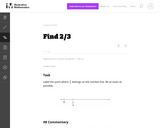Unrestricted Use
CC BY
Rating
0.0 stars

This simple-looking problem reveals much about how well students understand unit fractions as well as representing fractions on a number line.

Subject:
Mathematics
Numbers and Operations
Material Type:
Activity/Lab
Provider:
Illustrative Mathematics
Provider Set:
Illustrative Mathematics
Author:
Illustrative Mathematics
05/01/2012Rating
0.0 stars

Fractions on a number line including improper contains various videos, question answer, games and partner work are in this Roadmap. Also included is reteaching and an assessment.

Subject:
Mathematics
Material Type:
Activity/Lab
Assessment
Lesson
Author:
Monique Coulman
03/15/2019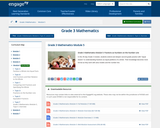Conditional Remix & Share Permitted
CC BY-NC-SA
Rating
0.0 stars

In this 35-day Grade 3 module, students extend and deepen second grade practice with "equal shares" to understanding fractions as equal partitions of a whole. Their knowledge becomes more formal as they work with area models and the number line.

Subject:
Mathematics
Ratios and Proportions
Material Type:
Module
Provider:
New York State Education Department
Provider Set:
EngageNY
02/03/2013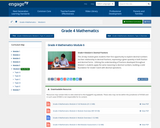Conditional Remix & Share Permitted
CC BY-NC-SA
Rating
0.0 stars

This 20-day module gives students their first opportunity to explore decimal numbers via their relationship to decimal fractions, expressing a given quantity in both fraction and decimal forms.  Utilizing the understanding of fractions developed throughout Module 5, students apply the same reasoning to decimal numbers, building a solid foundation for Grade 5 work with decimal operations.

Subject:
Mathematics
Ratios and Proportions
Material Type:
Module
Provider:
New York State Education Department
Provider Set:
EngageNY
02/01/2014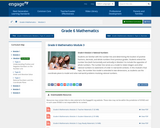Conditional Remix & Share Permitted
CC BY-NC-SA
Rating
0.0 stars

Students are familiar with the number line and determining the location of positive fractions, decimals, and whole numbers from previous grades. Students extend the number line (both horizontally and vertically) in Module 3 to include the opposites of whole numbers. The number line serves as a model to relate integers and other rational numbers to statements of order in real-world contexts. In this module's final topic, the number line model is extended to two-dimensions, as students use the coordinate plane to model and solve real-world problems involving rational numbers.

Subject:
Mathematics
Numbers and Operations
Material Type:
Module
Provider:
New York State Education Department
Provider Set:
EngageNY
10/20/2013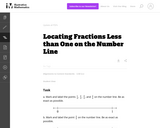Unrestricted Use
CC BY
Rating
0.0 stars

In this number line task students must treat the interval from 0 to 1 as a whole, partition the whole into the appropriate number of equal sized parts, and then locate the fraction(s).

Subject:
Mathematics
Numbers and Operations
Material Type:
Activity/Lab
Provider:
Illustrative Mathematics
Provider Set:
Illustrative Mathematics
Author:
Illustrative Mathematics